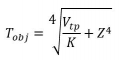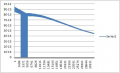# Curve fit for sensor equation

Thread Starter

#### Vindhyachal Takniki

Joined Nov 3, 2014
579
1. Have a thermistor, data whose dataset is available not anything else from company except B value. Attached is data.

2. Trying to make a equation for it and implement for 8 bit MCU, usin IAR IDE.

3. First I made this code, steinhart thermistor equation. calculated values of A,B,C from this site: https://www.thinksrs.com/downloads/programs/therm calc/ntccalibrator/ntccalculator.html . Put three values at -20C, 25C & 100C and get A,B,C

Code:
uint8_t get_ntc_temperature_4(float32_t resistance , float32_t *tempearture_c)
{
uint8_t err = 0U;
float32_t x;
float32_t x2;
float32_t x3;

if( (resistance <= 956040.0f) &&  (resistance >= 6683.0f) )
{
x = logf(resistance);
x2 = x * x;
x3 = x2 * x;
x = 0.000641295533f + (0.0002233488434f * x) + (0.0000000926090998f * x3);
x = 1.0f / x;
*tempearture_c = x - 273.15f;

}
else
{
err = 1U;
}

return err;

}

4. Then made equation using curve fit tool and below is code:

Code:
uint8_t get_ntc_temperature_2(float32_t resistance , float32_t *tempearture_c)
{
uint8_t err = 0U;
float32_t c0;
float32_t c1;
float32_t c2;

if( (resistance <= 956040.0f) &&  (resistance >= 482170.0f) )
{
c0 = 14.5029518320319131f;
c1 = -0.000057344817030149406f;
c2 = 0.000000000021788855747503695f;
}
else if(resistance >= 250140.0f)
{
c0 = 29.3136847463533279f;
c1 = -0.000119496884518122221f;
c2 = 0.0000000000876747221220605721f;
}
else if(resistance >= 130230.0f)
{
c0 = 45.3985586636038647f;
c1 = -0.000249401966454676711f;
c2 = 0.000000000352768556658954028f;
}
else if(resistance >= 71230.0f)
{
c0 = 62.1448935476119572f;
c1 = -0.000506560472110208432f;
c2 = 0.00000000135019658772898851f;
}
else if(resistance >= 39200.0f)
{
c0 = 79.7753263268828902f;
c1 = -0.00100556530593662709f;
c2 = 0.00000000491385322365418885f;
}
else if(resistance >= 21830.0f)
{
c0 = 98.8979251125107339f;
c1 = -0.00198872984475097995f;
c2 = 0.0000000176669521860767602f;
}
else if(resistance >= 12750.0f)
{
c0 = 118.809289117525665f;
c1 = -0.00381338043851388829f;
c2 = 0.0000000598289053823529209f;
}
else if(resistance >= 7327.0f)
{
c0 = 140.313484336487619f;
c1 = -0.00722784306211046376f;
c2 = 0.000000196440993753396293f;
}
else if(resistance >= 6683.0f)
{
c0 = 133.215333415477943f;
c1 = -0.00480709971651089099f;
c2 = 0.0f;
}
else
{
err = 1U;
}

if(0U == err)
{
*tempearture_c = c0 + (c1 * resistance) + (c2 * resistance * resistance);

}

return err;

}
5. Size build on full size optimization on IAR for 8 bit MCU:
a) No function call:
5 138 bytes of readonly code memory
139 bytes of readonly data memory
357 bytes of readwrite data memory

b) With polynomial equation fit:
5 593 bytes of readonly code memory
139 bytes of readonly data memory
357 bytes of readwrite data memory

c) With natural log:
5 820 bytes of readonly code memory
139 bytes of readonly data memory
359 bytes of readwrite data memory

6. Noticed that with polynomial fit extra space is 455 bytes & with natural log its 682 bytes.

7. Error with polynomial fit is +0.069 to -0.098C.
Error with natural log is slightly more though.

8. Any other better method to implement or curve fit this data? Max end function accuracy I am looking is +-0.099C.

PS: In function call, I got coefficients generated in float64_t in curve fit toolbox, put f in the end though.
I have enough time for float operations and circuit is main powered so no issues in processing time,

Thread Starter

#### Vindhyachal Takniki

Joined Nov 3, 2014
579
here is file

#### Attachments

• 13.4 KB Views: 3
Thread Starter

#### Vindhyachal Takniki

Joined Nov 3, 2014
579
My thermistor equation works ok mathematically. Now I want to fit equation for thermopile. PFA its data-sheet, excel data points and text file(which is tab separated values for area of interest around 38301 values).

In text file: We are able to measure :
a) Thermopile mV (column 3)
b) NTC temperature(column 2)
c) Need to find object temperature from above two variables.

So need to make equation: Z= F(x,y)
We asked the company manufacturer for equation here is what they have replied: "The table is built in 25 degree C. The others are generated via mathematical formula which is confidential."
So need to make equation on own.

Page 6 of pdf has a formula, but it doesn't fit the formula given. If we put Vtp, Tobj & Ta , K values are always different for any given data set.

I made the equation: its third order equation contains 10 coefficients with x & y powers.
But had to divide entire thermopile range into 13 parts like : -50 to 7C , 7C to 58C and so on upto 700C. Each of these 13 sets have different 3 order coefficients to fit, within error range of +-0.09999.

This is purely mathematical model required here, anyone has idea on what is there any equation which fits as manufacturer mentions but didn't disclose.

#### Attachments

• 1,008.4 KB Views: 1
• 1.5 MB Views: 1
• 1.1 MB Views: 2

#### MisterBill2

Joined Jan 23, 2018
8,288
Thermistors are usually a poor choice for accurate temperature measurement at more than one point, because they are rather non-linear. They are OK for monitoring the difference between cold, cool, warm, and hot. The sellers will tell you that accuracy is possible, and certainly the curve can be accurate at one point, and fairly close nearby. That is the reason that you get a data table and not a formula.

#### MrChips

Joined Oct 2, 2009
23,114
Rule #1 - I never use float in embedded MCU systems. It is too inefficient and not a necessity.

Rule #2 - Ignore resistance. That is just an unnecessary step.

Collect a sequence of data pairs that covers your intended temperature range. Record temperature vs MCU reading (ADC reading if that is what you are using). 10 to 20 data pairs would be nice.

Post your data and I can see what I can do.

What is your temperature range and accuracy you desire?

#### Papabravo

Joined Feb 24, 2006
15,549
•ci139

#### MrAl

Joined Jun 17, 2014
8,073
My thermistor equation works ok mathematically. Now I want to fit equation for thermopile. PFA its data-sheet, excel data points and text file(which is tab separated values for area of interest around 38301 values).

In text file: We are able to measure :
a) Thermopile mV (column 3)
b) NTC temperature(column 2)
c) Need to find object temperature from above two variables.

So need to make equation: Z= F(x,y)
We asked the company manufacturer for equation here is what they have replied: "The table is built in 25 degree C. The others are generated via mathematical formula which is confidential."
So need to make equation on own.

Page 6 of pdf has a formula, but it doesn't fit the formula given. If we put Vtp, Tobj & Ta , K values are always different for any given data set.

I made the equation: its third order equation contains 10 coefficients with x & y powers.
But had to divide entire thermopile range into 13 parts like : -50 to 7C , 7C to 58C and so on upto 700C. Each of these 13 sets have different 3 order coefficients to fit, within error range of +-0.09999.

This is purely mathematical model required here, anyone has idea on what is there any equation which fits as manufacturer mentions but didn't disclose.
Do i understand that you are done with the thermistor and are now moving on to the other device?

What accuracy do you need (usually stated in percent, not an absolute value)?

Thread Starter

#### Vindhyachal Takniki

Joined Nov 3, 2014
579
I am using thermopile which has thermistor in-built into it. Here its datasheet also.

This formula doesn't exactly fit in dataset given.For example:
1. If I take set no 1 from text file attached in my previous post.
Tobj = -50+273.15K
NTC = 0+ 273.15K
Vtp = -2.90303987541749/1000 volts
values of K comes out to be K : 9.40360645303949000000E-13

2. If I take set no 61 from text file
Tobj = -49+273.15K
NTC = 9+ 273.15K
Vtp = -3.5222018314122336/1000 volts
values of K comes out to be K : 9.23698403345936000000E-13

2. If I take set no 8053 from text file
Tobj = 107+273.15K
NTC = 45+ 273.15K
Vtp = 8.260617415613451/1000 volts
values of K comes out to be K : 7.76451812140848000000E-13

Similarly I tried plotting graph of K calculated from all 38301 values given: this is what I get for first 32000 values graph(excel limitation)As seen value of K keeps on falling for given dataset.
I have attached excel also on which I had calculated K values.
Those falling to zero are actually diviide by zero error, where Tobj & Tamb equals each other.

#### Attachments

• 1.5 MB Views: 0
• 3.4 MB Views: 0

#### MrAl

Joined Jun 17, 2014
8,073
First, not everyone can read .xlsx files you should really avoid that and use plain text or something else.

Second, you may be over reaching on this. The accuracy you are after may not be repeatable. Maybe you can take a few small data sets with the same operating conditions on different days with different humidity levels and see if you see a variation there.

For an example, i have used thermistors in the past for various home and professional projects. One was a military project, another was a simple refrigerator monitor.
In the refrigerator monitor i used the regular natural log function that describes thermistors that contains Just three constants and was able to get good results using the standard formula for thermistors. That is probably because the accuracy and repeatability did not have to be too perfect.
Another application was an outdoor thermometer. Had to read from -20 to 110 degrees F. Again, didnt have to be perfect so the key was to just use a 1 percent thermistor.
The military project was a measuring meter. The meter had to be accurate over a wide temperature range and the basic movement could not be replaced due to the military constraint for the type of product it goes into. So the thermistor was used to temperature compensate the meter movement. It had to be put in an oven and tested over the range of temperatures then adjusted, then retested, etc. But the accuracy of the entire meter only had to be maybe 2 percent. So again it did not have to be super accurate.

The equation i used is called (i just found out the name) the:
Steinhart–Hart equation.

The question is, do you have any information that tells you the kind of accuracy you are after is actually attainable in real life?

Last edited:

#### MisterBill2

Joined Jan 23, 2018
8,288
The simple approach will be to graph all of the points in the data table in a smooth graph, and then for each interval of resistance record the temperature in another data table, and then the thermistor value drives a lookup function to display the actual temperature. No sensor equation needed. And no 15 place math required. It could be done with an 8-bit system. And when the thermistor changes with age, probably still able to be used.

#### MrAl

Joined Jun 17, 2014
8,073
(Accidentally posted a second time somehow, ignore this one)